# Three blocks and a spring on an inclined plane...

Gold Member

## Homework Statement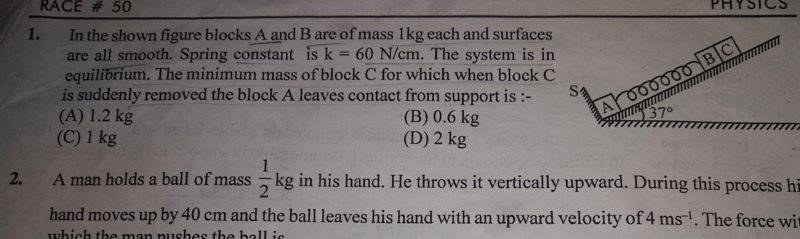See question number 1.

## Homework Equations

Work Energy Theorem,
work done by all the forces=change in K.E.

## The Attempt at a Solution

I tried solving this question this way,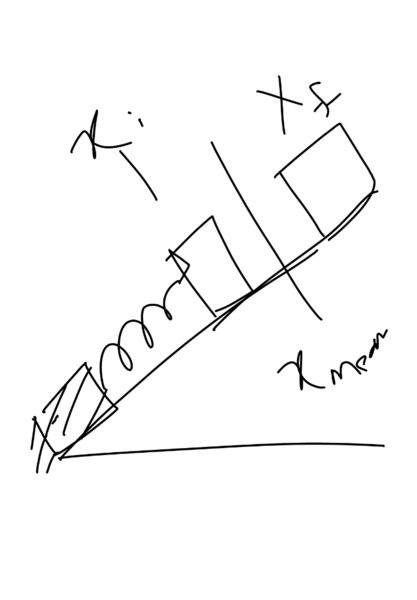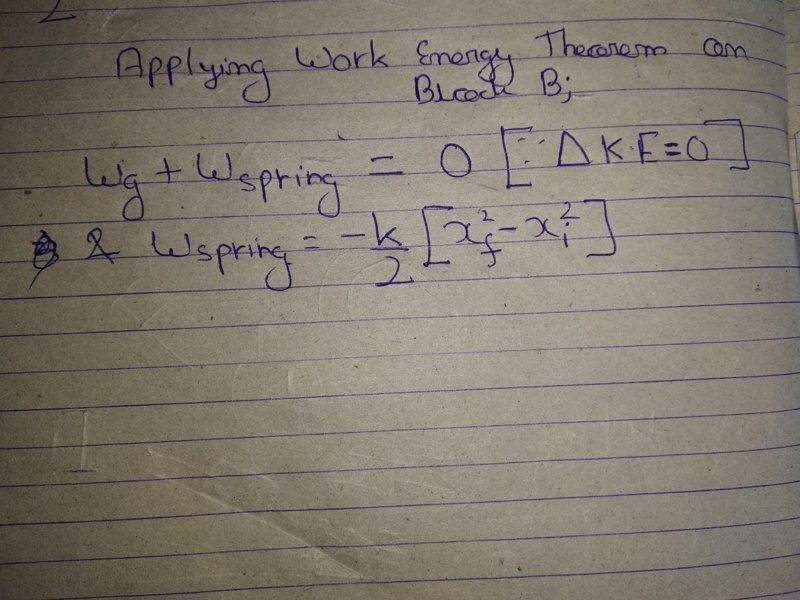I will be thankful for any help!

#### Attachments

Last edited by a moderator:

haruspex
Homework Helper
Gold Member
It might be simpler to start from the final situation. When A just loses contact, what is the tension in the spring?

Gold Member
It would be,
-0.6x=1*10*sin(37)
So what's next?
It might be simpler to start from the final situation. When A just loses contact, what is the tension in the spring?

haruspex
Homework Helper
Gold Member
It would be,
-0.6x=1*10*sin(37)
So what's next?
Actually I asked for the tension, but you went a step further and found the extension.
So what is the total energy in the system at that point?

Gold Member
It would be zero,Right?
Actually I asked for the tension, but you went a step further and found the extension.
So what is the total energy in the system at that point?

haruspex
Homework Helper
Gold Member
It would be zero,Right?
You have three components to consider: spring PE, GPE and KE. They are not all zero.

Gold Member
I think I need to apply the work energy theorem.
But on which block ?
You have three components to consider: spring PE, GPE and KE. They are not all zero.

rude man
Homework Helper
Gold Member
In particular, what do you think KE might be if mC is minimum mass to just lose contact?
(Interesting problem. The answer is independent of no less than two parameters that you'd think at first blush would matter.).

haruspex
Homework Helper
Gold Member
I think I need to apply the work energy theorem.
That comes later. First try to say what the total energy is at the end. Take the initial height of block B as your zero for GPE. Invent variables as necessary for unknowns.

Gold Member
That comes later. First try to say what the total energy is at the end. Take the initial height of block B as your zero for GPE. Invent variables as necessary for unknowns.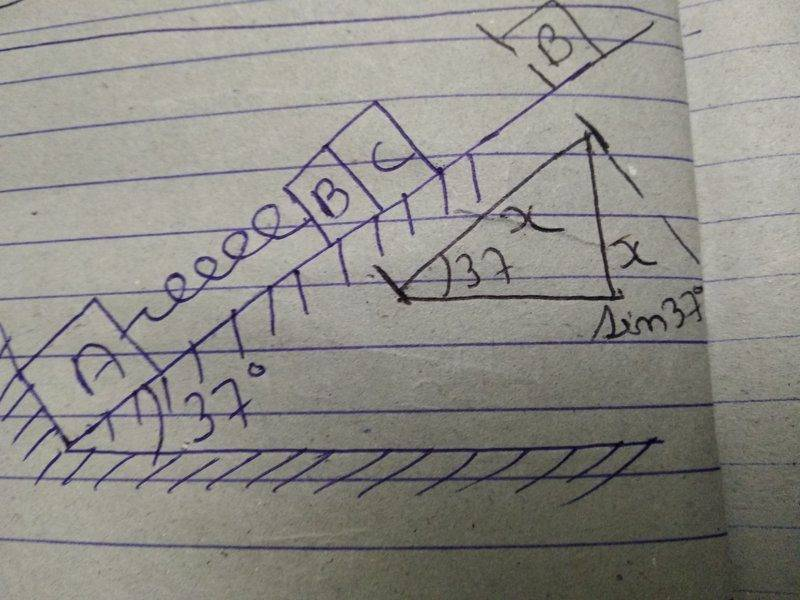I tried solving it this way this time...,
I have formed two equations,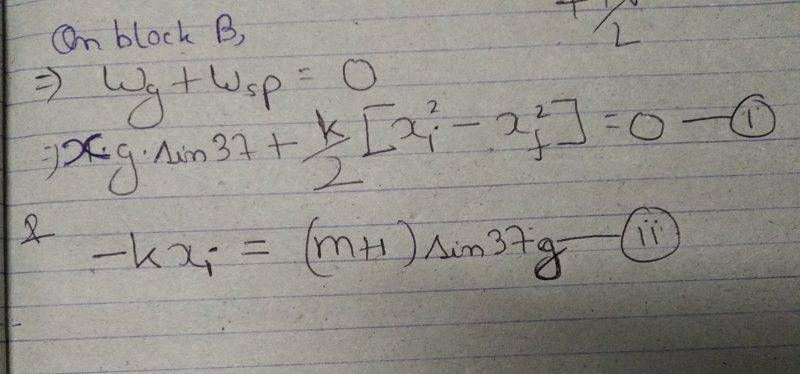Are these two equations useful in finding "m"?
Or,do I need to find something more?
I will be thankful for help!

#### Attachments

haruspex
Homework Helper
Gold Member
View attachment 230083
I tried solving it this way this time...,
I have formed two equations,
View attachment 230087
Are these two equations useful in finding "m"?
Or,do I need to find something more?
I will be thankful for help!
In eqn (1),your first term is missing a factor. It does not appear to have the dimension of energy.
What is the relationship between the x on the left and xi and xj on the right?

In eqn (2), you seem to have "mH" (or m+1?), but I do not know what this is supposed to be. And are you sure about that minus sign?

Gold Member
In eqn (1),your first term is missing a factor. It does not appear to have the dimension of energy.
What is the relationship between the x on the left and xi and xj on the right?

In eqn (2), you seem to have "mH" (or m+1?), but I do not know what this is supposed to be. And are you sure about that minus sign?
In the first equation W_gravity on B=1*x*g*sin(37),is it correct now?
And in equation 2
It's not "mH",It's (m+1)[sorry!For my poor handwriting]
Yes I think there would be a minus sign because the formula for spring force is "-k*x".
Is it correct?

haruspex
Homework Helper
Gold Member
In the first equation W_gravity on B=1*x*g*sin(37),is it correct now?
And in equation 2
It's not "mH",It's (m+1)[sorry!For my poor handwriting]
Ok, you fooled me in both because you left out the units. When you use a symbol like m for mass you do not need to state units because m has dimension, but when you plug in a specific value like 1kg that's what you must write, 1kg, not just "1".
Yes I think there would be a minus sign because the formula for spring force is "-k*x".
That's fine if you are taking xi as negative.
But you did not answer my other question: what is the relationship between the xi and xj you have in the second equation and the x you have in the first equation?

Gold Member
But you did not answer my other question: what is the relationship between the xi and xj you have in the second equation and the x you have in the first equation?
The relation between xi, xj and x would be "xi+xj=x".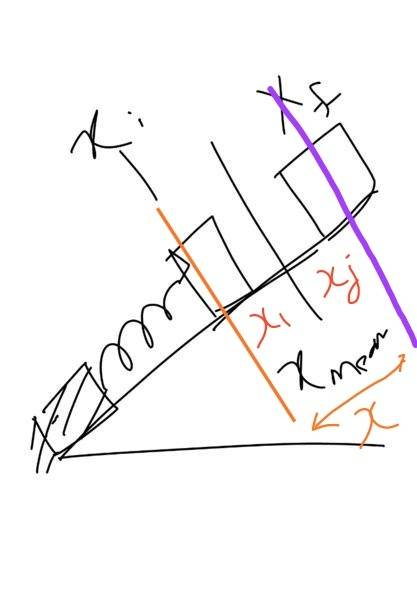Please tell how to proceed further!